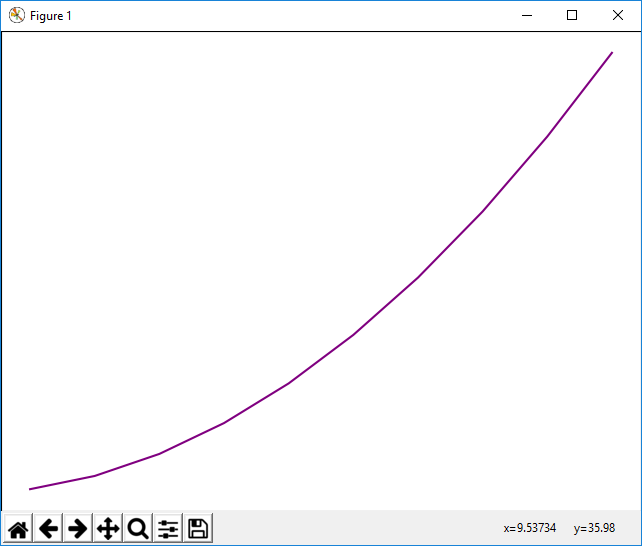﻿ How to Change the Color of a Graph Plot in Matplotlib with Python ﻿# How to Change the Color of a Graph Plot in Matplotlib with PythonIn this article, we show how to change the color of a graph plot in matplotlib with Python.

So when you create a plot of a graph, by default, matplotlib will choose a color for you.

However, you may have a certain color you want the plot to be.

Matplotlib allows you to specify the color of the graph plot.

This is done with the color attribute.

The color attribute is specified with the plot() function, when you are plotting the graph.

This is shown in the following code below.

So the first thing we have to do is import matplotlib. We do this with the line, import matplotlib.pyplot as plt

We then create a variable fig, and set it equal to, plt.figure()

This creates a figure object, which of course is initially empty, because we haven't populated it with anything. We then add axes to this figure. We then have our x coordinates that range from 0 to 10.

We then plot the square of the x coordinates.

It is with the plot() function that we specify the color of the plot. In this case, we set the color of the graph plot equal to purple.

However, you can set this to any other color, including green, red, yellow, etc.

You can also look up the hex code for a color and specify this hex code. Just when you put in the hex code, precede it with a hashtag (#).

Lastly, we show the figure with the show() function.

This works if you're using a python IDE other than jupyter notebooks. If you are using jupyter notebooks, then you would not use, plt.show(). Instead you would specify in the code right after importing matplotlib, %matplotlib inline

This line allows the figure of a graph to be shown with jupyter notebooks.

After running the following code above, we get the following figure with the graph plot being purple shown in the image below.So now you see a figure object with a graph plot that is a purple color.

And this is how you can change the color of graph plot in matplotlib with Python.

Related Resources

How to Randomly Select From or Shuffle a List in Python